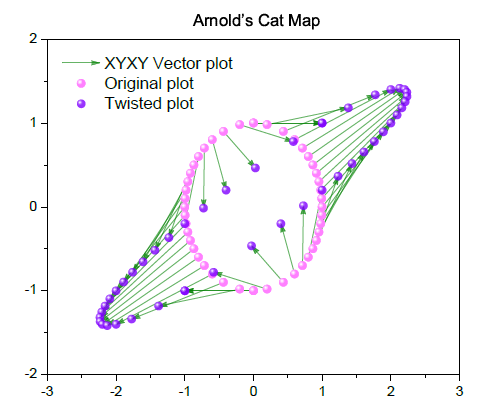Skip Navigation Links.

### Sketch of Arnold's cat mapping transformationDescription:

In mathematics, Arnold's cat map is a chaotic map from the torus into itself. This graph is simulating the effect after distortion with Arnold's cat map (only one calculation), the new (Xn+1,Yn+1) is calculated from (Xn, Yn) according to: Xn+1=2Xn+Yn; Yn+1=Xn+Yn.

This graph includes both the original shape and the twisted shape together with the 2D XYXY vector plot. The vectors are generated from starting points (from original shape) and ending points (from twisted shape), and are used here to visualize the effect of distortion of this transformation.